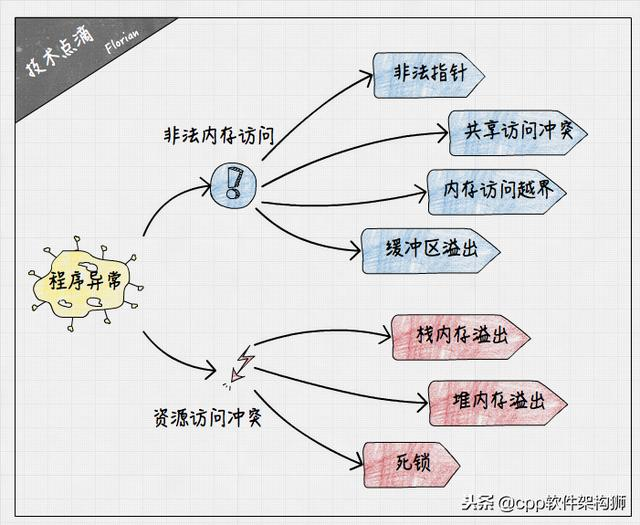﻿ 内存溢出，死锁怎么办？教你如何排查_丝画阁(homeforexchange.cn)

## 内存溢出，死锁怎么办？教你如何排查1. 非法内存访问（读/写）：非法指针、多线程共享数据访问冲突、内存访问越界、缓冲区溢出等。
2. 资源访问冲突：栈内存溢出、堆内存溢出、死锁等。

# 一、非法内存访问

1. 非法指针``` long *ptr;
*ptr = 0; // 空指针
ptr = (long *)0x12345678;
*ptr = 100; // 非法地址访问
```

2. 多线程共享数据访问冲突

``` // 全局变量
long *ptr = (long *)malloc(sizeof(long));
// 线程1
if (ptr) {
*ptr = 100; // 潜在的非法地址访问
}
// 线程2
free(ptr);
ptr = NULL;
```3. 内存访问越界

``` void out_of_bound() {
long *ptr;
long buffer[] = {0};
ptr = buffer;
buffer = 0; // 越界访问导致ptr被覆盖
ptr++;
}
`````` void backScanArray(long buffer[]) {
for(unsigned int index = sizeof(buffer) / sizeof(long) - 1;
index > 0; index--) {
printf("%ld
", buffer[index]);
}
}
```

4. 缓冲区溢出

``` void stack_over_flow() {
long *ptr;
long buffer[] = {0};
ptr = buffer;
buffer = 0; // 缓冲区溢出攻击
ptr++;
}
```# 二、资源访问冲突

1. 栈内存溢出```\$ ulimit -s
8192
```

```void left_recursive() {
left_recursive();
}
```

2. 堆内存溢出C语言使用malloc/free尽享堆内存的申请和释放，开发者编写程序时，必须小心翼翼地控制这两对函数的调用逻辑，以防申请和释放不对等诱发内存泄漏问题。而实际开发过程中，人工保证这样的准确性是十分困难的，后边我们会介绍如何使用分析工具帮我们排查程序中潜在的内存漏洞问题。

3. 死锁# 三、程序异常解决方法

1. CoreDumped异常分析

step 1:

```\$ sudo ulimit -c unlimited
\$ sudo echo core > /proc/sys/kernel/core_pattern
```

step 2:

``` void out_of_bound() {
long *ptr;
long buffer[] = {0};
ptr = buffer;
buffer = 0; // 越界访问导致ptr被覆盖
ptr++;
}

void main() {
out_of_bound();
}
```

step 3:

```\$ gcc main.c -o main -g
\$ ./main
Segmentation fault (core dumped)
\$ ls core.*
core.9251
```

step 4:

```\$ gdb main core.9251
./x: line 4: 9251 Segmentation fault (core dumped) ./main
[New LWP 9251]
Core was generated by `./main'.
Program terminated with signal SIGSEGV, Segmentation fault.
#0 0x00000000004004d6 in out_of_bound () at main.c:6
6 ptr++;
(gdb) backtrace
#0 0x00000000004004d6 in out_of_bound () at main.c:6
#1 0x00000000004004f5 in main () at main.c:10
(gdb) print ptr
\$1 = (long *) 0x0
(gdb)
```

gdb输出了程序崩溃时代码的执行位置，main.c文件的第6行。使用backtrace命令可以打印当时的函数调用栈信息，以方便定位出错的上层调用逻辑。使用print命令打印ptr指针的值，确实为0，与我们之前的讨论一致。上面分析仅仅是一个非常简单的示例，实际开发过程中遇到的出错位置可能更隐蔽，甚至是库函数的二进制代码，届时需要根据个人经验来具体问题具体分析了。

2. 使用Valgrind进行内存泄漏和死锁检测

Valgrind是非常强大的内存调试、内存泄漏检测以及性能分析工具，它可以模拟执行用户二进制程序，帮助用户分析潜在的内存泄漏和死锁的可能逻辑。

step 1:

```\$ wget http://valgrind.org/downloads/valgrind-3.12.0.tar.bz2
\$ tar xf valgrind-3.12.0.tar.bz2
\$ cd valgrind-3.12.0
\$ ./configure --prefix=/usr/local/
\$ make && sudo make install
\$ valgrind --version
valgrind-3.12.0
```

step 2:

```#include
void main() {
malloc(4);
}
```

step 3:

```\$ valgrind --tool=memcheck --leak-check=full ./main
==24470== Memcheck, a memory error detector
==24470== Copyright (C) 2002-2015, and GNU GPL'd, by Julian Seward et al.
==24470== Using Valgrind-3.12.0 and LibVEX; rerun with -h for copyright info
==24470== Command: ./main
==24470==
==24470==
==24470== HEAP SUMMARY:
==24470== in use at exit: 4 bytes in 1 blocks
==24470== total heap usage: 1 allocs, 0 frees, 4 bytes allocated
==24470==
==24470== LEAK SUMMARY:
==24470== definitely lost: 0 bytes in 0 blocks
==24470== indirectly lost: 0 bytes in 0 blocks
==24470== possibly lost: 0 bytes in 0 blocks
==24470== still reachable: 4 bytes in 1 blocks
==24470== suppressed: 0 bytes in 0 blocks
==24470== Reachable blocks (those to which a pointer was found) are not shown.
==24470== To see them, rerun with: --leak-check=full --show-leak-kinds=all
==24470==
==24470== For counts of detected and suppressed errors, rerun with: -v
==24470== ERROR SUMMARY: 0 errors from 0 contexts (suppressed: 0 from 0)
```

step 4:

```#include
#include
#include
void *run1(void *args)
{
sleep(1);
}
void *run2(void *args)
{
sleep(1);
}
void main()
{
if (pthread_create(&tid, NULL, &run1, NULL) != 0)
{
exit(1);
}
if (pthread_create(&tid, NULL, &run2, NULL) != 0)
{
exit(1);
}
}
```

step 5:

```\$ gcc main.c -o main -g -lpthread
\$ valgrind --tool=helgrind ./main
==24652== Helgrind, a thread error detector
==24652== Copyright (C) 2007-2015, and GNU GPL'd, by OpenWorks LLP et al.
==24652== Using Valgrind-3.12.0 and LibVEX; rerun with -h for copyright info
==24652== Command: ./main
==24652==
Ctrl + C
==24652==
==24652== Process terminating with default action of signal 2 (SIGINT)
==24652== by 0x400806: main (main.c:39)
==24652==
==24652== at 0x51427AE: clone (in /usr/lib/libc-2.24.so)
==24652== by 0x4007BA: main (main.c:30)
==24652==
==24652== ----------------------------------------------------------------
==24652==
==24652== at 0x4E4CF1C: __lll_lock_wait (in /usr/lib/libpthread-2.24.so)
==24652== by 0x4C2EE18: mutex_lock_WRK (hg_intercepts.c:894)
==24652== by 0x40073F: run1 (main.c:12)
==24652==
==24652==
==24652== at 0x51427AE: clone (in /usr/lib/libc-2.24.so)
==24652== by 0x4007E7: main (main.c:34)
==24652==
==24652== ----------------------------------------------------------------
==24652==
==24652== at 0x4E4CF1C: __lll_lock_wait (in /usr/lib/libpthread-2.24.so)
==24652== by 0x4C2EE18: mutex_lock_WRK (hg_intercepts.c:894)
==24652== by 0x400780: run2 (main.c:21)
==24652==
==24652==
==24652== For counts of detected and suppressed errors, rerun with: -v
==24652== Use --history-level=approx or =none to gain increased speed, at
==24652== the cost of reduced accuracy of conflicting-access information
==24652== ERROR SUMMARY: 2 errors from 2 contexts (suppressed: 1 from 1)
```

# 参考资料

1. coredump简介与coredump原因总结: http://blog.csdn.net/newnewman80/article/details/8173770
2. C语言申请内存时堆栈大小限制: http://blog.csdn.net/xxxxxx91116/article/details/10068555